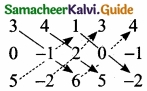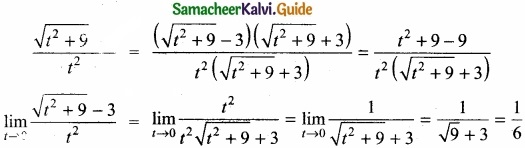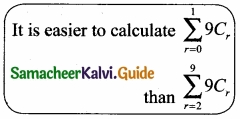Students can Download Tamil Nadu 11th Maths Model Question Paper 1 English Medium Pdf, Tamil Nadu 11th Maths Model Question Papers helps you to revise the complete Tamilnadu State Board New Syllabus, helps students complete homework assignments and to score high marks in board exams.

## TN State Board 11th Maths Model Question Paper 1 English Medium

General Instructions:

1. The question paper comprises of four parts.
2. You are to attempt all the parts. An internal choice of questions is provided wherever applicable.
3. All questions of Part I, II, III and IV are to be attempted separately.
4. Question numbers 1 to 20 in Part I are Multiple Choice Questions of one mark each.
These are to be answered by choosing the most suitable answer from the given four alternatives and writing the option code and the corresponding answer
5. Question numbers 21 to 30 in Part II are two-mark questions. These are to be answered in about one or two sentences.
6. Question numbers 31 to 40 in Part III are three-mark questions. These are to be answered in above three to five short sentences.
7. Question numbers 41 to 47 in Part IV are five-mark questions. These are to be answered in detail Draw diagrams wherever necessary.

Time: 2:30 Hours
Maximum Marks: 90

PART – 1

I. Choose the correct answer. Answer all the questions: [20 × 1 = 20]

Question 1.
For non empty sets A and B if A ⊂ B then (A × B) n (B × A) is equal to ………………….
(a) A ∩ B
(b) A × A
(c) B × B
(d) none of these
(b) A × AQuestion 2.
The solution set of the inequality |x – 1| ≥ |x – 3| is ………………..
(a) [0, 2]
(b) [2, ∞)
(c) (0, 2)
(d) (-∞, 2)
(b) [2, ∞)

Question 3.
The numer of solutions of x2 + |x – 1| = 1 is …………………
(a) 1
(b) 0
(c) 2
(d) 3
(c) 2Question 4.
Which of the following is not true?
(a) sin θ = – $$\frac{3}{4}$$
(b) cos θ = -1
(c) tan θ = 25
(d) sec θ = $$\frac{1}{4}$$
(d) sec θ = $$\frac{1}{4}$$

Question 5.
Let fk(x) = $$\frac{1}{k}$$ [sink x + cos2 x] where x ∈ R and k ≥ 1. Then f4(x) – f6(x) = …………………
(a) $$\frac{1}{4}$$
(b) $$\frac{1}{12}$$
(c) $$\frac{1}{6}$$
(d) $$\frac{1}{3}$$
(b) $$\frac{1}{12}$$Question 6.
If A and B are coefficients of xn in the expansion of (1 + x)2n and (1 + x)2n-1 respectively then $$\frac{A}{B}$$ =
(a) $$\frac{1}{2}$$
(b) $$\frac{1}{n}$$
(c) 1
(d) 2
(d) 2

Question 7.
The value of 15C8 + 15C9 – 15C6 – 15C7 is …………………
(a) 0
(b) 1
(c) 2
(d) 3
(a) 0Question 8.
The slope of the line which makes an angle 45° with the line 3x -y = – 5 are …………………
(a) 1, -1
(b) $$\frac{1}{2}$$, -2
(c) 1, $$\frac{1}{2}$$
(d) 2, $$\frac{-1}{2}$$
(b) $$\frac{1}{2}$$, -2

Question 9.
The sum of the binomial co-efficients is ………………….
(a) 2n
(b) 2n
(c) n2
(d) 1
(b) 2nQuestion 10.
If the square of the matrix $$\begin{pmatrix} \alpha & \beta \\ \gamma & -\alpha \end{pmatrix}$$ satisfy the relation
(a) 1 + α2 + βγ = 0
(b) 1 – α2 – βγ = 0
(c) 1 – α2 + βγ = 0
(d) 1 + α2 – βγ = 0
(b) 1 – α2 – βγ = 0

Question 11.
The value of x for which the matrix A = $$\begin{bmatrix} e^{ x-2 } & e^{ 7+x } \\ e^{ 2+x } & e^{ 2x+3 } \end{bmatrix}$$ is singular is ………………….
(a) 9
(b) 8
(c) 7
(d) 6
(b) 8Question 12.
If A = $$\begin{pmatrix} \lambda & 1 \\ -1 & -\lambda \end{pmatrix}$$ then for what value of λ, A2 = 0?
(a) 0
(b) ±1
(c) -1
(d) 1
(b) ±1

Question 13.
limx→1 $$\frac { xe^{ x }-sinx }{ x }$$ is ……………….
(a) 1
(b) 2
(c) 3
(d) 0
(d) 0

Question 14.
If the points whose position vectors 10$${ \vec { i } }$$ + 3$${ \vec { j } }$$ + 12$${ \vec { i } }$$ – 5$${ \vec { j } }$$ and a$${ \vec { i } }$$ + 11$${ \vec { j } }$$ are collinear then the value of a is ……………….
(a) 3
(b) 5
(c) 6
(d) 8
(d) 8

Question 15.
If y = mx + c and f(0) = f'(0) = 1 then f(2) = ………………..
(a) 1
(b) 2
(c) 3
(d) 4
(c) 3Question 16.
limx→1 $$\frac { e^{ x }-e }{ x-1 }$$ = ……………….
(a) 1
(b) e
(c) ∞
(d) 0
(b) e

Question 17.
∫23x+5 dx is ………………..
(a) $$\frac { 3(2^{ 3x+5 }) }{ log2 }$$ + c
(b) $$\frac { 2^{ 3x+5 } }{ 2log(3x+5) }$$ + c
(c) $$\frac { 2^{ 3x+5 } }{ 2log3 }$$ + c
(d) $$\frac { 2^{ 3x+5 } }{ 3log2 }$$ + c
(d) $$\frac { 2^{ 3x+5 } }{ 3log2 }$$ + c

Question 18.
∫x2 cos xdx is ………………..
(a) x2sinx + 2xcosx – 2 sinx + c
(b) x2 – 2xcosx – 2sinx + c
(c) -x2 + 2xcosx + 2sinx + c
(d) -x2 – 2xcosx + 2 sinx + c
(a) x2sinx + 2xcosx – 2 sinx + cQuestion 19.
∫$$\frac { x }{ 1+x^{ 2 } }$$ dx = ………………
(a) tan-1 x + c
(b) log (1 + x2) + c
(c) log x + c
(d) $$\frac{1}{2}$$ log (1 + x2) + c
(d) $$\frac{1}{2}$$ log (1 + x2) + c

Question 20.
Ten coins are tossed. The probability of getting atleast 8 heads is …………….
(a) $$\frac{7}{64}$$
(b) $$\frac{7}{32}$$
(c) $$\frac{7}{128}$$
(d) $$\frac{7}{16}$$
(c) $$\frac{7}{128}$$

PART – II

II. Answer any seven questions. Question No. 30 is compulsory. [7 × 2 = 14]

Question 21.
To secure an A grade one must obtain an average of 90 marks or more in 5 subjects each of maximum 100 marks. If A scored 84, 87, 95, 91 in first four subjects, what is the minimum mark be scored in the fifth subject to get an A grade in the course?
Required marks = 5 × 90 = 450
Total marks obtained in 4 subjects = 84 + 87 + 95 + 91 = 357
So required marks in the fifth subject = 450 – 357 = 93.Question 22.
Prove that (1 + tan1°) (1 + tan2°) (1 + tan 3°) …. (1 + tan 44°) is a multiple of 4?
45°= 1 + 44 (or) 2 + 43 (or) 3 + 42 (or) 22 + 23
So we have 22 possible pairs
sa the product is (2) (22) = 44
which is ÷ by 4

Question 23.
Find the distinct permutations of the letters of the word MISSISSIPPI?
MISSISSIPPI
Number of letters = 11
Here M-1 time, I-4timcs, S-4times, P-2timcs
So total number of arrangement is of this word = $$\frac { 11! }{ 4!4!2! }$$
$$\frac { 11\times 10\times 9\times 8\times 7\times 6\times 5\times 4! }{ 4\times 3\times 2\times 1\times 2\times 1\times 4! }$$ = 34650Question 24.
Compare |A| using Sarrus rule if A = $$\left[\begin{array}{ccc} 3 & 4 & 1 \\ 0 & -1 & 2 \\ 5 & -2 & 6 \end{array}\right]$$|A| = [3(-1) (6) + 4(2)(5) + 1(0)(-2)] -[5(-1)(1) + (-2)(2)3 + 6(0)(4)] = [-18 + 40 + 0] – [-5 – 12 + 0] = 22 + 17 = 39.

Question 25.
If |$${ \vec { a } }$$|, |$${ \vec { b } }$$| = 6, |$${ \vec { c } }$$| =7 and $${ \vec { a } }$$ + $${ \vec { b } }$$ + $${ \vec { c } }$$ = 0 find $${ \vec { a } }$$.$${ \vec { b } }$$ + $${ \vec { b } }.$$ $${ \vec { c } }$$ + $${ \vec { c } }$$.$${ \vec { a } }$$
Given $$\bar { a }$$+$$\bar { b }$$+$$\bar { c }$$ = 0
⇒ ($$\bar { a }$$+$$\bar { b }$$+$$\bar { c }$$)2 = 0
(i.e;) |$$\bar { a }$$|2 + |$$\bar { b }$$|2 + |$$\bar { c }$$|2 + 2 [$$\bar { a }$$.$$\bar { b }$$+$$\bar { b }$$.$$\bar { c }$$+$$\bar { c }$$.$$\bar { a }$$) = 0
⇒ 52+62+72+2($$\bar { a }$$.$$\bar { b }$$+$$\bar { b }$$.$$\bar { c }$$+$$\bar { c }$$.$$\bar { a }$$) = 0
⇒2($$\bar { a }$$.$$\bar { b }$$+$$\bar { b }$$.$$\bar { c }$$+$$\bar { c }$$.$$\bar { a }$$) = – 25 – 36 – 49 = -110
⇒$$\bar { a }$$.$$\bar { b }$$+$$\bar { b }$$.$$\bar { c }$$+$$\bar { c }$$.$$\bar { a }$$ = $$\frac{-110}{2}$$ = -55Question 26.
Find $$\lim _{ t\rightarrow 0 }{ \frac { \sqrt { t^{ 2 }+9-3 } }{ t^{ 2 } } }$$
We can’t apply the quotient Iieorem it.:iiediatcly. Use the algebra technique of rationalising the numerator.Question 27.
Evaluate y = e-x.log x?
y = e-x log x = uv(say)
Here u = e-x and v = log x
⇒ u’ = -e-x and v’ = uv’ + vu’
Now y = uv ⇒y’ = uv’ + vu’
(i.e). $$\frac{dy}{dx}$$ = e-x($$\frac{1}{x}$$) + log x(- e-x)
= e-x($$\frac{1}{x}$$ – log x)Question 28.
Evaluate ∫$$\frac { 1 }{ \sqrt { 1+(4x)^{ 2 } } }$$ dx
Let I = ∫$$\frac { 1 }{ \sqrt { 1+4x^{ 2 } } }$$dx = ∫$$\frac { 1 }{ \sqrt { 1+(2x^{ 2 }) } }$$ dx
Putting 2x = t ⇒ 2 dx = dt ⇒ dx = $$\frac{1}{2}$$ dt
Thus, I = $$\frac{1}{2}$$∫$${ \frac { 1 }{ \sqrt { 1^{ 2 }+t^{ 2 } } } }$$ dt
I = $$\frac{1}{2}$$ log |t+$$\sqrt { t^{ 2 }+1 }$$ + c = $$\frac{1}{2}$$ log |2x + $$\sqrt { (2x)^{ 2 }+1 }$$| + c
I = $$\frac{1}{2}$$ log |2x+$$\sqrt { 4^{ 2 }+1 }$$| + c.

Question 29.
Nine coins are tossed once. Find the probablity to get atleast 2 heads?
Let S be the sample and A be the event of getting at least two heads.
Therefore, the event $$\bar { A }$$ denotes, getting at most one head.
n(S) = 29 = 521, n($$\bar { A }$$) = 9C0 + 9C1 = 1 + 9 = 10
P($$\bar { A }$$) = $$\frac{10}{512}$$ = $$\frac{5}{256}$$
P(A) = 1- P($$\bar { A }$$) = 1 – $$\frac{5}{256}$$ = $$\frac{251}{256}$$Question 30.
If P(A) denotes the power set of A, then find n(P(P(ϕ))))?
Since P(∅) contains 1 element, P(P(∅)) contains 21 elements and hence P(P(P(∅))) contains 22 elements. That is, 4 elements.

PART – III

III. Answer any seven questions. Question No. 40 is compulsory. [7 × 3 = 21]

Question 31.
A simple cipher takes a number and codes it, using the function f(x) = 3x – 4. Find the inverse of this function, determine whether the inverse is also a function and verify the symmetrical property about the line y = x (by drawing the lines)?

Question 32.
Show that 4 sin A sin (60° + A). sin(60° – A) = sin 3A?Question 33.
How many strings of length 6 can be formed using letters of the word FLOWER if (i) either starts with F or ends with R?

Question 34.
Find the distance of the line 4x – y = 0 from the point P(4, 1) measured along the line making an angle 135° with the positive x- axis?Question 35.
Using factor theorem prove that $$\left|\begin{array}{lll} x+1 & 3 & 5 \\ 2 & x+2 & 5 \\ 2 & 3 & x+4 \end{array}\right|$$ = (x – 1)2 + ( x+ 9)

Question 36.
Find the unit vectors perpendicular to each of the vectors $${ \vec { a } }$$ + $${ \vec { b } }$$ and $${ \vec { a } }$$ – $${ \vec { b } }$$. Where $${ \vec { a } }$$ = $${ \vec { i } }$$ + $${ \vec { j } }$$ + $${ \vec { k } }$$ and b = $${ \vec { i } }$$ + 2$${ \vec { j } }$$ + 3$${ \vec { k } }$$?Question 37.
If y = tan-1 $$\left(\frac{1+x}{1-x}\right)$$, find y’?

Question 38.
Evaluate ∫$$\frac { x }{ \sqrt { 1+x^{ 2 } } }$$ dx?

Question 39.
If A and B are two events such that P(A∪B) = 0.7, P(A∩B) = 0.2 and P(B) = 0.5 then show that A and B are independent?Question 40.
Resolve into partial fractions $$\frac { 2x^{ 2 }+5x-11 }{ x^{ 2 }+2x-3 }$$

PART – IV

IV. Answer all the questIons [7 × 5 = 35]

Question 41.
(a) A photocopy store charges Rs. 1.50 per copy for the first 10 copies and Rs. 1.00 per copy after the 10th copy. Let x be the number of copies, and let y be the total cost of photocopying.
(a) Draw graph of the cost as x goes from 0 to 50 copies?
(b) Find the cost of making 40 copies

[OR]

(b) If the difference of the roots of the equation 2x2 – (a + 1)x + a – 1 = 0 is equal to their product then prove that a =2?Question 42.
(a) Prove that tan 70° – tan 20° – 2 tan 40° = 4 tan 10°?

[OR]

(b) In a ∆ABC, if a = 2$$\sqrt{3}$$, b = 2$$\sqrt{2}$$ and C = 75° find the other side and the angles?

Question 43.
(a) Use induction to prove that n3 – 7n + 3, is divisible by 3, for all natural numbers n?

[OR]

(b) Evaluate y = (x2 + 1) $$\sqrt [ 3 ]{ x^{ 2 }+2 }$$?Question 44.
(a) Show that f(x) f(y) = f(x + y), where f(x) = $$\left[\begin{array}{ccc} \cos x & -\sin x & 0 \\ \sin x & \cos x & 0 \\ 0 & 0 & 1 \end{array}\right]$$

[OR]

(b) The chances of X, Y and Z becoming managers of a certain company are 4 : 2 : 3. The probabilities that bonus scheme will be introduced if X, Y and Z become managers are 0.3, 0.5 and 0.4 respectively. 1f the bonus scheme has been introduced, what is the probability that Z was appointed as the manager?Question 45.
(a) If the equation λx2 – 10xy + 12y2 + 5x – 16y – 3 = O represents a pair of straight lines, find

1. The value of λ and the separate equations of the lines
2. Angle between the lines

[OR]

(b) Show that

1. $$\lim _{n \rightarrow \infty} \frac{1+2+3+\ldots+n}{3 n^{2}+7 n+2}=\frac{1}{6}$$
2. $$\lim _{n \rightarrow \infty} \frac{1^{2}+2^{2}+\ldots+(3 n)^{2}}{(1+2+\ldots+5 n)(2 n+3)}=\frac{9}{25}$$
3. $$\lim _{n \rightarrow \infty} \frac{1}{1.2}+\frac{1}{2.3}+\frac{1}{3.4}+\ldots .+\frac{1}{n(n+1)}=1$$Question 46.
(a) A man repays an amount of ₹3250 by paying ₹20 in the first month and then increases the payment by ₹15 per month. How long will it take him to clear the amount?

[OR]

(b) Find the area of the triangle whose vertices are A (3, -1, 2), B (1, -1, -3) and C (4, -3, 1)?Question 47.
(a) Evaluate (x + 1) $$\sqrt { 2x+3 }$$?
[OR]

(b) Evaluate ∫cosec2x dx?